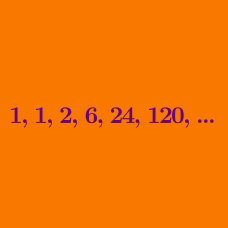Number Theory

# Factorials Warmup

Which of the following is equal to $\Large \frac{6!}{6}$?

What is the smallest positive integer $n$ such that $n!$ has exactly 1 trailing zero?

Note: Trailing zeros are sequences of zeros that come at the end of a number. For example, 1,000 has 3 trailing zeros and 1,001 has no trailing zeros.

What is the smallest value of $n$ such that $n!$ is divisible by $9?$

Note: If $n$ is a positive integer, $n! = 1 \times 2 \times 3 \times \cdots \times (n - 1) \times n.$ For example, $5! = 1 \times 2 \times 3 \times 4 \times 5.$

Are there 3 consecutive positive integers whose product is not divisible by $3! \, ?$

$x! = 3! \times 5!$ What is $x?$

×